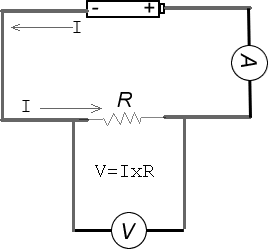####Circuit Fundamentals

A circuit is a closed path for electron (current) flow

A circuit must have four key elements

1.  A voltage source (The battery)

2. A load  (R - The resistor)

3. A complete path

4. A switch

Voltage is the force in a voltage source and is measured in VOLTS.

The symbol for voltage is “V”.  Voltage is measured with an instrument called Voltmenter (V), as illustrated in the circuit above.

An example of a voltage source is a battery.

Current is (how fast) the measure of the flow of electricity in a circuit and it is measured in Amps.
“Amps” is a short form for Amperes  (symbol A - in the diagram above you see an Ammeter - an instrument used to measure current).

The symbol for Current is “I”.

Loads can be generally defined as the resistance to the flow of current in a circuit.

Resistance is the opposition to current flow.

The symbol for resistance is “R”.
A resistor is a small electronic component used to add resistance (or load) in a circuit.
Resistor values are given in ohms

W - this is the symbol for Greek letter "omega